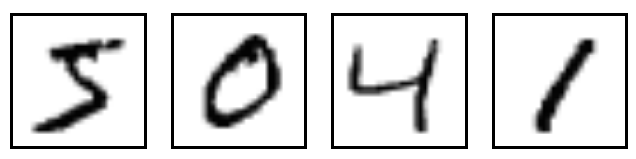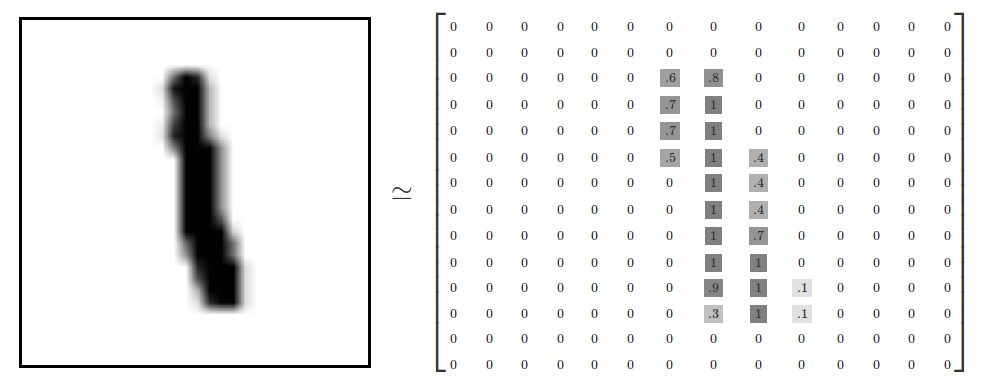#2017 iT 邦幫忙鐵人賽
DAY 3
2
Big Data

## 目標

• 下載並熟悉 MNIST 資料集
• 建立 tensorflow softmax regression model
• 訓練 model 並計算出準確度

``````import numpy as np
import tensorflow as tf
import matplotlib.pyplot as plt
%matplotlib inline
``````## 下載並熟悉 MNIST data

MNIST 資料集的官網是在Yann LeCun's website．這裡我們只要在 python 內把以下的兩行程式碼貼上，就可以下載 MNIST 的資料集．

``````from tensorflow.examples.tutorials.mnist import input_data
mnist = input_data.read_data_sets("MNIST_data/", one_hot=True)
``````
``````Extracting MNIST_data/train-images-idx3-ubyte.gz
Extracting MNIST_data/train-labels-idx1-ubyte.gz
Extracting MNIST_data/t10k-images-idx3-ubyte.gz
Extracting MNIST_data/t10k-labels-idx1-ubyte.gz
``````

MNIST 資料集分成三個部分

1. 55,000 筆的 training data (`mnist.train`)
2. 10,000 筆的 test data (`mnist.test`)
3. 5,000 筆的 validation data (`mnist.validation`)

``````# 來看看 mnist 的型態
print type(mnist)
print mnist.train.num_examples
print mnist.validation.num_examples
print mnist.test.num_examples
``````
``````<class 'tensorflow.contrib.learn.python.learn.datasets.base.Datasets'>
55000
5000
10000
``````
``````print("讓我們看一下 MNIST 訓練還有測試的資料集長得如何")
train_img = mnist.train.images
train_label = mnist.train.labels
test_img = mnist.test.images
test_label = mnist.test.labels
print
print(" train_img 的 type : %s" % (type(train_img)))
print(" train_img 的 dimension : %s" % (train_img.shape,))
print(" train_label 的 type : %s" % (type(train_label)))
print(" train_label 的 dimension : %s" % (train_label.shape,))
print(" test_img 的 type : %s" % (type(test_img)))
print(" test_img 的 dimension : %s" % (test_img.shape,))
print(" test_label 的 type : %s" % (type(test_label)))
print(" test_label 的 dimension : %s" % (test_label.shape,))
``````
``````讓我們看一下 MNIST 訓練還有測試的資料集長得如何

train_img 的 type : <type 'numpy.ndarray'>
train_img 的 dimension : (55000, 784)
train_label 的 type : <type 'numpy.ndarray'>
train_label 的 dimension : (55000, 10)
test_img 的 type : <type 'numpy.ndarray'>
test_img 的 dimension : (10000, 784)
test_label 的 type : <type 'numpy.ndarray'>
test_label 的 dimension : (10000, 10)
````````````trainimg = mnist.train.images
trainlabel = mnist.train.labels
nsample = 1
randidx = np.random.randint(trainimg.shape, size=nsample)

for i in [0, 1, 2]:
curr_img   = np.reshape(trainimg[i, :], (28, 28)) # 28 by 28 matrix
curr_label = np.argmax(trainlabel[i, :] ) # Label
plt.matshow(curr_img, cmap=plt.get_cmap('gray'))
plt.title("" + str(i + 1) + "th Training Data "
+ "Label is " + str(curr_label))
``````tensorflow 學習筆記30

0